Elementary Algebra 2e

# 6.2Use Multiplication Properties of Exponents

Elementary Algebra 2e6.2 Use Multiplication Properties of Exponents

### Learning Objectives

By the end of this section, you will be able to:

• Simplify expressions with exponents
• Simplify expressions using the Product Property for Exponents
• Simplify expressions using the Power Property for Exponents
• Simplify expressions using the Product to a Power Property
• Simplify expressions by applying several properties
• Multiply monomials

### Be Prepared 6.4

Before you get started, take this readiness quiz.

Simplify: $34·34.34·34.$
If you missed this problem, review Example 1.68.

### Be Prepared 6.5

Simplify: $(−2)(−2)(−2).(−2)(−2)(−2).$
If you missed this problem, review Example 1.50.

### Simplify Expressions with Exponents

Remember that an exponent indicates repeated multiplication of the same quantity. For example, $2424$ means to multiply 2 by itself 4 times, so $2424$ means $2·2·2·22·2·2·2$.

Let’s review the vocabulary for expressions with exponents.

### Exponential Notation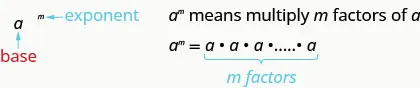This is read $aa$ to the $mthmth$ power.

In the expression $amam$, the exponent $mm$ tells us how many times we use the base $aa$ as a factor.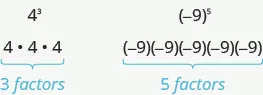Before we begin working with variable expressions containing exponents, let’s simplify a few expressions involving only numbers.

### Example 6.16

Simplify: $4343$ $7171$ $(56)2(56)2$ $(0.63)2.(0.63)2.$

### Try It 6.31

Simplify: $6363$ $151151$ $(37)2(37)2$ $(0.43)2.(0.43)2.$

### Try It 6.32

Simplify: $2525$ $211211$ $(25)3(25)3$ $(0.218)2.(0.218)2.$

### Example 6.17

Simplify: $(−5)4(−5)4$ $−54.−54.$

### Try It 6.33

Simplify: $(−3)4(−3)4$ $−34.−34.$

### Try It 6.34

Simplify: $(−13)2(−13)2$ $−132.−132.$

Notice the similarities and differences in Example 6.17 and Example 6.17 ! Why are the answers different? As we follow the order of operations in part the parentheses tell us to raise the $(−5)(−5)$ to the 4th power. In part we raise just the 5 to the 4th power and then take the opposite.

### Simplify Expressions Using the Product Property for Exponents

You have seen that when you combine like terms by adding and subtracting, you need to have the same base with the same exponent. But when you multiply and divide, the exponents may be different, and sometimes the bases may be different, too.

We’ll derive the properties of exponents by looking for patterns in several examples.

First, we will look at an example that leads to the Product Property.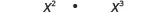What does this mean?How many factors altogether?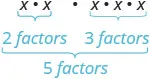So, we have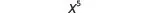Notice that 5 is the sum of the exponents, 2 and 3.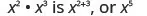We write:

$x2·x3x2+3x5x2·x3x2+3x5$

The base stayed the same and we added the exponents. This leads to the Product Property for Exponents.

### Product Property for Exponents

If $aa$ is a real number, and $mandnmandn$ are counting numbers, then

$am·an=am+nam·an=am+n$

To multiply with like bases, add the exponents.

An example with numbers helps to verify this property.

$22·23=?22+34·8=?2532=32✓22·23=?22+34·8=?2532=32✓$

### Example 6.18

Simplify: $y5·y6.y5·y6.$

### Try It 6.35

Simplify: $b9·b8.b9·b8.$

### Try It 6.36

Simplify: $x12·x4.x12·x4.$

### Example 6.19

Simplify: $25·2925·29$ $3·34.3·34.$

### Try It 6.37

Simplify: $5·555·55$ $49·49.49·49.$

### Try It 6.38

Simplify: $76·7876·78$ $10·1010.10·1010.$

### Example 6.20

Simplify: $a7·aa7·a$ $x27·x13.x27·x13.$

### Try It 6.39

Simplify: $p5·pp5·p$ $y14·y29.y14·y29.$

### Try It 6.40

Simplify: $z·z7z·z7$ $b15·b34.b15·b34.$

We can extend the Product Property for Exponents to more than two factors.

### Example 6.21

Simplify: $d4·d5·d2.d4·d5·d2.$

### Try It 6.41

Simplify: $x6·x4·x8.x6·x4·x8.$

### Try It 6.42

Simplify: $b5·b9·b5.b5·b9·b5.$

### Simplify Expressions Using the Power Property for Exponents

Now let’s look at an exponential expression that contains a power raised to a power. See if you can discover a general property.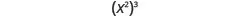What does this mean?How many factors altogether?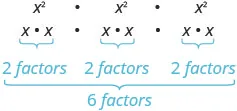So we haveNotice that 6 is the product of the exponents, 2 and 3.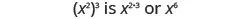We write:

$(x2)3x2·3x6(x2)3x2·3x6$

We multiplied the exponents. This leads to the Power Property for Exponents.

### Power Property for Exponents

If $aa$ is a real number, and $mandnmandn$ are whole numbers, then

$(am)n=am·n(am)n=am·n$

To raise a power to a power, multiply the exponents.

An example with numbers helps to verify this property.

$(32)3=?32·3(9)3=?36729=729✓(32)3=?32·3(9)3=?36729=729✓$

### Example 6.22

Simplify: $(y5)9(y5)9$ $(44)7.(44)7.$

### Try It 6.43

Simplify: $(b7)5(b7)5$ $(54)3.(54)3.$

### Try It 6.44

Simplify: $(z6)9(z6)9$ $(37)7.(37)7.$

### Simplify Expressions Using the Product to a Power Property

We will now look at an expression containing a product that is raised to a power. Can you find this pattern?

 $(2x)3(2x)3$ What does this mean? $2x·2x·2x2x·2x·2x$ We group the like factors together. $2·2·2·x·x·x2·2·2·x·x·x$ How many factors of 2 and of $xx$? $23·x323·x3$

Notice that each factor was raised to the power and $(2x)3(2x)3$ is $23·x323·x3$.

 We write: $(2x)3(2x)3$ $23·x323·x3$

The exponent applies to each of the factors! This leads to the Product to a Power Property for Exponents.

### Product to a Power Property for Exponents

If $aa$ and $bb$ are real numbers and $mm$ is a whole number, then

$(ab)m=ambm(ab)m=ambm$

To raise a product to a power, raise each factor to that power.

An example with numbers helps to verify this property:

$(2·3)2=?22·3262=?4·936=36✓(2·3)2=?22·3262=?4·936=36✓$

### Example 6.23

Simplify: $(−9d)2(−9d)2$ $(3mn)3.(3mn)3.$

### Try It 6.45

Simplify: $(−12y)2(−12y)2$ $(2wx)5.(2wx)5.$

### Try It 6.46

Simplify: $(5wx)3(5wx)3$ $(−3y)3.(−3y)3.$

### Simplify Expressions by Applying Several Properties

We now have three properties for multiplying expressions with exponents. Let’s summarize them and then we’ll do some examples that use more than one of the properties.

### Properties of Exponents

If $aandbaandb$ are real numbers, and $mandnmandn$ are whole numbers, then

 Product Property $am·an=am+nam·an=am+n$ Power Property $(am)n=am·n(am)n=am·n$ Product to a Power $(ab)m=ambm(ab)m=ambm$

All exponent properties hold true for any real numbers $mandnmandn$. Right now, we only use whole number exponents.

### Example 6.24

Simplify: $(y3)6(y5)4(y3)6(y5)4$ $(−6x4y5)2.(−6x4y5)2.$

### Try It 6.47

Simplify: $(a4)5(a7)4(a4)5(a7)4$ $(−2c4d2)3.(−2c4d2)3.$

### Try It 6.48

Simplify: $(−3x6y7)4(−3x6y7)4$ $(q4)5(q3)3.(q4)5(q3)3.$

### Example 6.25

Simplify: $(5m)2(3m3)(5m)2(3m3)$ $(3x2y)4(2xy2)3.(3x2y)4(2xy2)3.$

### Try It 6.49

Simplify: $(5n)2(3n10)(5n)2(3n10)$ $(c4d2)5(3cd5)4.(c4d2)5(3cd5)4.$

### Try It 6.50

Simplify: $(a3b2)6(4ab3)4(a3b2)6(4ab3)4$ $(2x)3(5x7).(2x)3(5x7).$

### Multiply Monomials

Since a monomial is an algebraic expression, we can use the properties of exponents to multiply monomials.

### Example 6.26

Multiply: $(3x2)(−4x3).(3x2)(−4x3).$

### Try It 6.51

Multiply: $(5y7)(−7y4).(5y7)(−7y4).$

### Try It 6.52

Multiply: $(−6b4)(−9b5).(−6b4)(−9b5).$

### Example 6.27

Multiply: $(56x3y)(12xy2).(56x3y)(12xy2).$

### Try It 6.53

Multiply: $(25a4b3)(15ab3).(25a4b3)(15ab3).$

### Try It 6.54

Multiply: $(23r5s)(12r6s7).(23r5s)(12r6s7).$

### Media

Access these online resources for additional instruction and practice with using multiplication properties of exponents:

### Section 6.2 Exercises

#### Practice Makes Perfect

Simplify Expressions with Exponents

In the following exercises, simplify each expression with exponents.

88.

$3535$ $9191$ $(13)2(13)2$ $(0.2)4(0.2)4$

89.

$104104$ $171171$ $(29)2(29)2$ $(0.5)3(0.5)3$

90.

$2626$ $141141$ $(25)3(25)3$ $(0.7)2(0.7)2$

91.

$8383$ $8181$ $(34)3(34)3$ $(0.4)3(0.4)3$

92.

$(−6)4(−6)4$ $−64−64$

93.

$(−2)6(−2)6$ $−26−26$

94.

$−(14)4−(14)4$ $(−14)4(−14)4$

95.

$−(23)2−(23)2$ $(−23)2(−23)2$

96.

$−0.52−0.52$ $(−0.5)2(−0.5)2$

97.

$−0.14−0.14$ $(−0.1)4(−0.1)4$

Simplify Expressions Using the Product Property for Exponents

In the following exercises, simplify each expression using the Product Property for Exponents.

98.

$d 3 · d 6 d 3 · d 6$

99.

$x 4 · x 2 x 4 · x 2$

100.

$n 19 · n 12 n 19 · n 12$

101.

$q 27 · q 15 q 27 · q 15$

102.

$45·4945·49$ $89·889·8$

103.

$310·36310·36$ $5·545·54$

104.

$y·y3y·y3$ $z25·z8z25·z8$

105.

$w5·ww5·w$ $u41·u53u41·u53$

106.

$w · w 2 · w 3 w · w 2 · w 3$

107.

$y · y 3 · y 5 y · y 3 · y 5$

108.

$a 4 · a 3 · a 9 a 4 · a 3 · a 9$

109.

$c 5 · c 11 · c 2 c 5 · c 11 · c 2$

110.

$m x · m 3 m x · m 3$

111.

$n y · n 2 n y · n 2$

112.

$y a · y b y a · y b$

113.

$x p · x q x p · x q$

Simplify Expressions Using the Power Property for Exponents

In the following exercises, simplify each expression using the Power Property for Exponents.

114.

$(m4)2(m4)2$ $(103)6(103)6$

115.

$(b2)7(b2)7$ $(38)2(38)2$

116.

$(y3)x(y3)x$ $(5x)y(5x)y$

117.

$(x2)y(x2)y$ $(7a)b(7a)b$

Simplify Expressions Using the Product to a Power Property

In the following exercises, simplify each expression using the Product to a Power Property.

118.

$(6a)2(6a)2$ $(3xy)2(3xy)2$

119.

$(5x)2(5x)2$ $(4ab)2(4ab)2$

120.

$(−4m)3(−4m)3$ $(5ab)3(5ab)3$

121.

$(−7n)3(−7n)3$ $(3xyz)4(3xyz)4$

Simplify Expressions by Applying Several Properties

In the following exercises, simplify each expression.

122.

$(y2)4·(y3)2(y2)4·(y3)2$ $(10a2b)3(10a2b)3$

123.

$(w4)3·(w5)2(w4)3·(w5)2$ $(2xy4)5(2xy4)5$

124.

$(−2r3s2)4(−2r3s2)4$ $(m5)3·(m9)4(m5)3·(m9)4$

125.

$(−10q2p4)3(−10q2p4)3$ $(n3)10·(n5)2(n3)10·(n5)2$

126.

$(3x)2(5x)(3x)2(5x)$ $(5t2)3(3t)2(5t2)3(3t)2$

127.

$(2y)3(6y)(2y)3(6y)$ $(10k4)3(5k6)2(10k4)3(5k6)2$

128.

$(5a)2(2a)3(5a)2(2a)3$ $(12y2)3(23y)2(12y2)3(23y)2$

129.

$(4b)2(3b)3(4b)2(3b)3$ $(12j2)5(25j3)2(12j2)5(25j3)2$

130.

$(25x2y)3(25x2y)3$ $(89xy4)2(89xy4)2$

131.

$(2r2)3(4r)2(2r2)3(4r)2$ $(3x3)3(x5)4(3x3)3(x5)4$

132.

$(m2n)2(2mn5)4(m2n)2(2mn5)4$ $(3pq4)2(6p6q)2(3pq4)2(6p6q)2$

Multiply Monomials

In the following exercises, multiply the monomials.

133.

$( 6 y 7 ) ( −3 y 4 ) ( 6 y 7 ) ( −3 y 4 )$

134.

$( −10 x 5 ) ( −3 x 3 ) ( −10 x 5 ) ( −3 x 3 )$

135.

$( −8 u 6 ) ( −9 u ) ( −8 u 6 ) ( −9 u )$

136.

$( −6 c 4 ) ( −12 c ) ( −6 c 4 ) ( −12 c )$

137.

$( 1 5 f 8 ) ( 20 f 3 ) ( 1 5 f 8 ) ( 20 f 3 )$

138.

$( 1 4 d 5 ) ( 36 d 2 ) ( 1 4 d 5 ) ( 36 d 2 )$

139.

$( 4 a 3 b ) ( 9 a 2 b 6 ) ( 4 a 3 b ) ( 9 a 2 b 6 )$

140.

$( 6 m 4 n 3 ) ( 7 m n 5 ) ( 6 m 4 n 3 ) ( 7 m n 5 )$

141.

$( 4 7 r s 2 ) ( 14 r s 3 ) ( 4 7 r s 2 ) ( 14 r s 3 )$

142.

$( 5 8 x 3 y ) ( 24 x 5 y ) ( 5 8 x 3 y ) ( 24 x 5 y )$

143.

$( 2 3 x 2 y ) ( 3 4 x y 2 ) ( 2 3 x 2 y ) ( 3 4 x y 2 )$

144.

$( 3 5 m 3 n 2 ) ( 5 9 m 2 n 3 ) ( 3 5 m 3 n 2 ) ( 5 9 m 2 n 3 )$

Mixed Practice

In the following exercises, simplify each expression.

145.

$( x 2 ) 4 · ( x 3 ) 2 ( x 2 ) 4 · ( x 3 ) 2$

146.

$( y 4 ) 3 · ( y 5 ) 2 ( y 4 ) 3 · ( y 5 ) 2$

147.

$( a 2 ) 6 · ( a 3 ) 8 ( a 2 ) 6 · ( a 3 ) 8$

148.

$( b 7 ) 5 · ( b 2 ) 6 ( b 7 ) 5 · ( b 2 ) 6$

149.

$( 2 m 6 ) 3 ( 2 m 6 ) 3$

150.

$( 3 y 2 ) 4 ( 3 y 2 ) 4$

151.

$( 10 x 2 y ) 3 ( 10 x 2 y ) 3$

152.

$( 2 m n 4 ) 5 ( 2 m n 4 ) 5$

153.

$( −2 a 3 b 2 ) 4 ( −2 a 3 b 2 ) 4$

154.

$( −10 u 2 v 4 ) 3 ( −10 u 2 v 4 ) 3$

155.

$( 2 3 x 2 y ) 3 ( 2 3 x 2 y ) 3$

156.

$( 7 9 p q 4 ) 2 ( 7 9 p q 4 ) 2$

157.

$( 8 a 3 ) 2 ( 2 a ) 4 ( 8 a 3 ) 2 ( 2 a ) 4$

158.

$( 5 r 2 ) 3 ( 3 r ) 2 ( 5 r 2 ) 3 ( 3 r ) 2$

159.

$( 10 p 4 ) 3 ( 5 p 6 ) 2 ( 10 p 4 ) 3 ( 5 p 6 ) 2$

160.

$( 4 x 3 ) 3 ( 2 x 5 ) 4 ( 4 x 3 ) 3 ( 2 x 5 ) 4$

161.

$( 1 2 x 2 y 3 ) 4 ( 4 x 5 y 3 ) 2 ( 1 2 x 2 y 3 ) 4 ( 4 x 5 y 3 ) 2$

162.

$( 1 3 m 3 n 2 ) 4 ( 9 m 8 n 3 ) 2 ( 1 3 m 3 n 2 ) 4 ( 9 m 8 n 3 ) 2$

163.

$( 3 m 2 n ) 2 ( 2 m n 5 ) 4 ( 3 m 2 n ) 2 ( 2 m n 5 ) 4$

164.

$( 2 p q 4 ) 3 ( 5 p 6 q ) 2 ( 2 p q 4 ) 3 ( 5 p 6 q ) 2$

#### Everyday Math

165.

Email Kate emails a flyer to ten of her friends and tells them to forward it to ten of their friends, who forward it to ten of their friends, and so on. The number of people who receive the email on the second round is $102102$, on the third round is $103103$, as shown in the table below. How many people will receive the email on the sixth round? Simplify the expression to show the number of people who receive the email.

Round Number of people
1 10
2 $102102$
3 $103103$
6 ?
166.

Salary Jamal’s boss gives him a 3% raise every year on his birthday. This means that each year, Jamal’s salary is 1.03 times his last year’s salary. If his original salary was $35,000, his salary after 1 year was $35,000(1.03)35,000(1.03)$, after 2 years was $35,000(1.03)235,000(1.03)2$, after 3 years was $35,000(1.03)335,000(1.03)3$, as shown in the table below. What will Jamal’s salary be after 10 years? Simplify the expression, to show Jamal’s salary in dollars. Year Salary 1 $35,000(1.03)35,000(1.03)$ 2 $35,000(1.03)235,000(1.03)2$ 3 $35,000(1.03)335,000(1.03)3$ 10 ? 167. Clearance A department store is clearing out merchandise in order to make room for new inventory. The plan is to mark down items by 30% each week. This means that each week the cost of an item is 70% of the previous week’s cost. If the original cost of a sofa was$1,000, the cost for the first week would be $1,000(0.70)1,000(0.70)$ and the cost of the item during the second week would be $1,000(0.70)21,000(0.70)2$. Complete the table shown below. What will be the cost of the sofa during the fifth week? Simplify the expression, to show the cost in dollars.

Week Cost
1 $1,000(0.70)1,000(0.70)$
2 $1,000(0.70)21,000(0.70)2$
3
4
5 ?
168.

Depreciation Once a new car is driven away from the dealer, it begins to lose value. Each year, a car loses 10% of its value. This means that each year the value of a car is 90% of the previous year’s value. If a new car was purchased for \$20,000, the value at the end of the first year would be $20,000(0.90)20,000(0.90)$ and the value of the car after the end of the second year would be $20,000(0.90)220,000(0.90)2$. Complete the table shown below. What will be the value of the car at the end of the eighth year? Simplify the expression, to show the value in dollars.

Year Cost
1 $20,000(0.90)20,000(0.90)$
2 $20,000(0.90)220,000(0.90)2$
3
8 ?

#### Writing Exercises

169.

Use the Product Property for Exponents to explain why $x·x=x2x·x=x2$.

170.

Explain why $−53=(−5)3−53=(−5)3$ but $−54≠(−5)4−54≠(−5)4$.

171.

Jorge thinks $(12)2(12)2$ is 1. What is wrong with his reasoning?

172.

Explain why $x3·x5x3·x5$ is $x8x8$, and not $x15x15$.

#### Self Check

After completing the exercises, use this checklist to evaluate your mastery of the objectives of this section.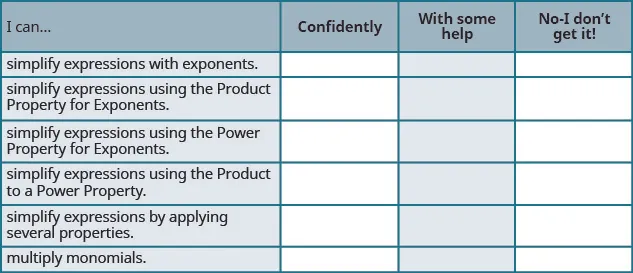After reviewing this checklist, what will you do to become confident for all goals?

Order a print copy

As an Amazon Associate we earn from qualifying purchases.